# Derivative of differentiable function satisfies intermediate value property

(diff) ← Older revision | Latest revision (diff) | Newer revision → (diff)

## Name

The result is sometimes called Darboux's theorem, and is attributed to the mathematician Darboux. However, there is another more famous theorem named after Darboux.

## Statement

### Endpoint one-sided version

Suppose$f$ is a function defined on an interval$[a,b]$. Suppose:

•$f'$ (the derivative of$f$) exists on the open interval$(a,b)$.
• The right hand derivative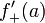$f'_+(a)$ exists
• The left hand derivative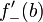$f'_-(b)$ exists

Suppose further that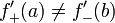$f'_+(a) \ne f'_-(b)$ and$w$ is a real number strictly between these two numbers. Then, there exists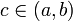$c \in (a,b)$ such that$f'(c) = w$.

### Two-sided version

Suppose$f$ is a differentiable function on an open interval$(p,q)$. Then, the derivative$f'$ satisfies the intermediate value property on$(p,q)$: for$a < b$, both in$(p,q)$, and any value$w$ is strictly between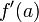$f'(a)$ and$f'(b)$, there exists$c \in (a,b)$ such that$f'(c) = w$.

## Proof

### Proof idea

The proof idea is to find a difference quotient that takes the desired value intermediate between$f'(a)$ and$f'(b)$, then use Fact (3).

### Proof details for one-sided endpoint version using the mean value theorem

The two-sided version follows from the one-sided endpoint version, so we only prove the latter.

Given:$f$ is a function defined on an interval$[a,b]$. Suppose:

•$f'$ (the derivative of$f$) exists on the open interval$(a,b)$.
• The right hand derivative$f'_+(a)$ exists
• The left hand derivative$f'_-(b)$ exists

Further,$f'_+(a) \ne f'_-(b)$ and$w$ is a real number strictly between these two numbers.

To prove: There exists$c \in (a,b)$ such that$f'(c) = w$.

Proof: Consider the function$g$ defined on the interval$[0,1]$ as follows. By$\Delta f$ we denote the difference quotient. Note that we use$f'_+$ for the right hand derivative and$f'_-$ for the left hand derivative:$g(t) := \left\lbrace\begin{array}{rrl} f'_+(a), & & t = 0 \\ (\Delta f)(2tb + a(1 - 2t),a) = & \frac{f(2tb + a(1 - 2t)) - f(a)}{2t(b - a)}, & 0 < t < 1/2 \\ (\Delta f)(b,a) = & \frac{f(b) - f(a)}{b - a}, & t = 1/2\\ (\Delta f)(b,(2 - 2t)a + b(2t - 1)) = & \frac{f(b) - f((2 - 2t)a + b(2t - 1))}{(2 - 2t)(b - a)}, & 1/2 < t < 1\\ f'_-(b), & & t = 1 \\\end{array}\right.$

Note that for$0 < t < 1$,$g(t)$ is a difference quotient between two points in$[a,b]$, at least one of which is one of the endpoints$a,b$.

Step no. Assertion/construction Facts used Given data used Previous steps used Explanation
1$g$ is continuous on$(0,1/2)$ and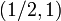$(1/2,1)$. Fact (1)$f$ is differentiable on$(a,b)$ and one-sided differentiable at the endpoints. Follows directly from continuity of$f$ and the nature of the expressions.
2$g$ is right continuous at$t = 0$ definition of derivative as a limit of a difference quotient [SHOW MORE]
3$g$ is continuous at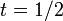$t = 1/2$ Fact (1)$f$ is differentiable We take the limit by plugging$t = 1/2$ in the left side and right side definition and check. We again use the continuity of$f$ by Fact (1).
4$g$ is left continuous at$t = 1$ definition of derivative as a limit of a difference quotient [SHOW MORE]
5$g$ is continuous on$[0,1]$. Steps (1)-(4) step-combination direct.
6 There exists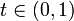$t \in (0,1)$ such that$g(t) = w$. In particular$w$ is a difference quotient between two points, both of them in$[a,b]$. Fact (2)$w$ is between$f'(a)$ and$f'(b)$. Step (5) By definition,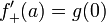$f'_+(a) = g(0)$ and$f'_-(b) = g(1)$. Step (5) and Fact (1) tell us that since$w$ is between these, there exists$t \in (0,1)$ such that$g(t) = w$.
7 There exists$c \in (a,b)$ such that$f'(c) = w$. Fact (3)$f$ is differentiable on$(a,b)$ Step (6) Step-fact combination direct.

### Proof details for direct proof of one-sided version

There is a direct proof that does not involve any appeal to the mean value theorem. This proof is shorter, but relies on the extreme value theorem. Note that the previous proof that relies on the mean value theorem indirectly relies on the extreme value theorem, whereas the proof below makes a direct appeal to the extreme value theorem.

Fill this in later